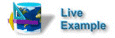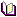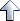Application Techniques

# Using Tree Controls

How to use the tree control on a form.

Details

Category

DescriptionYou can run this technique code from:

NOTE   First make sure that database is running on your localhost SilverStream ServerRelated reading

See the chapter on the Form Designer in the Tools Guide

# Creating and loading the tree controlThe following code creates and loads a tree control into a list box control called tcExample. The code uses the `add()` method to create a root node with three child nodes, each of which has three grandchild nodes. It also creates an object array that stores each node ID and the parent node ID.

This code is in the formActivate event:

```  // select the first item
cmbxRelationship.setSelectedIndex(0);
// Create the array of objects used to store the user data
Object objNodes[] = new Object;
objNodes = "0"; // Root node has no parent, so set to "0"
objNodes = "0"; // Root node is the first, so set id to "0"
AgoTreeControlNode nodeRoot = tcExample.add (null, 0, "Root Node",
objNodes, null);
objNodes, null); // Add three child nodes to the root node
for (int i = 1; i < 4; i++)
{
// Set the user data for the child node
objNodes = new Object;
objNodes = "0";  // Parent of all child nodes is 0
// Use child counter i to determine id
// for the new child node
objNodes = String.valueOf(i);
nodeRoot, AgcTreeControl.CHILD, "Child " + i, objNodes,
null);
// Increment the id counter
m_id++;

// Add three child nodes to each child (GrandChild)
for (int j = 1; j < 4; j++)
{
// Set the user data for the grand child node
objNodes = new Object;
// Use child counter i to determine
// the parent id for the new child node
objNodes = String.valueOf(i);
// Use grandchild counter j to determine
// the id for the new child node
objNodes = String.valueOf(i * 3 + j);

AgoTreeControlNode nodeGrandChild =
tcExample.add (nodeChild, 1, "GrandChild " +
i + "," + j, objNodes, null);
// Increment the id counter
m_id++;
}
}
```

• The AgoTreeControlNode object is a node of an AgcTreeControl. This object is returned after a new item is added to a tree control, allowing you to add new items after or under it, or to delete it later.

• The `add()` method is declared as follows:

```  AgoTreeControlNode add(AgoTreeControlNode relativeTo, int relationship,
String text,Object userData, Image image)
```

# Expanding a node on the tree controlThe following code uses `expandNode()` with `getSelectedNode()`to expand the currently selected node in the tree control. In this example, the tree is contained in a list box control named tcExample.

```  // Expand the currently-selected node.
tcExample.expandNode(tcExample.getSelectedNode());
```

• The `expand()` node method takes a node object as an argument. In this example, the object is returned by the method `getSelectedNode()`.

# Collapsing a node on the tree controlThe following code uses the `collapseNode()` method with the` getSelectedNode()` method to collapse the currently selected node.

```  // Collapse the currently-selected node
tcExample.collapseNode(tcExample.getSelectedNode());
```

• The` collapseNode()` method takes a node object as an argument. The object is returned by `getSelectedNode()`.

# Getting and setting text on a selected nodeThe following code first uses` getSelectedNode()` to get the currently selected node in tcExample. It then uses the `getText()` method to get the text label for the selected node, and` setText()` to place the text in the text field fldText.

```  // Get the selected node.
AgoTreeControlNode nodeSelected = tcExample.getSelectedNode();

//If we don't have a valid node, return
if (nodeSelected == null) return;

// Get the text from selected node and display in fldGetText
fldText.setText(nodeSelected.getText());
```

• The AgoTreeControlNode object is a node of an AgcTreeControl. It is returned when a tree item is selected so that you can retrieve the item's text or argument.

• The node object is contained in the list box tcExample. The method `getSelectedNode()` returns the currently selected node which is stored as nodeSelected.

• The `getText()` method gets the string label for the selected node, and passes this string to `setText()`

# Adding a node to the tree controlThe following code uses the tree control's `add()` method to add a new node entered in the text field fldadd to the currently selected node. It determines the relationship of the new node to the selected node by getting the value entered in the combo box cmbxRelationship. The code also stores the ID of the parent node and the sequential number for each node added.

```  private void handle_btnAdd_actionPerformed(ActionEvent evt)
{
lblMessage.setText("");

// If no node selected, return
AgoTreeControlNode nodeSelected = tcExample.getSelectedNode();
if (nodeSelected == null)
{
lblMessage.setText(MSG_SELECT_NODE);
return;
}

// If no node name entered, return
lblMessage.setText(MSG_ENTER_NODE_NAME );
return;
}
// Get the value from cmbxRelationship to determine
// how the new row will be added:
// if no relationship specified, return.
//            1 = CHILD
//            2 = NEXT
//            3 = PREVIOUS
//            4 = LAST
String sValue = (String) cmbxRelationship.getValue();
if (sValue == null)
{
lblMessage.setText(MSG_ENTER_RELATIONSHIP);
return;
}
int iRelationship = Integer.parseInt(sValue);

// Get the user data from the selected node
Object[] userData = (Object[]) nodeSelected.getUserData();

// Get the selected node's parent id
String sParentNode = (String) userData;
// Get the selected node's id
String sNodeID = (String) userData;

// Re-create the object array
userData = new Object;

// If we are adding a child node, use the selected
// node's id as the parent id of the new node,
// otherwise use the selected node's parent id
if (iRelationship == 1)
userData = sNodeID;
else
userData = sParentNode;

// Increment the id counter
m_id++;

// Use the new value of the id counter as the id
// of the new node
userData = "" + m_id;

// Add the new node according to the relationship selected
userData, null);

// Clear the node name from the data-entry field
}

```

• The AgoTreeControlNode object is a node of an AgcTreeControl. This object is returned after a new item is added to a tree control, allowing you to add new items after or under it, or to delete it later.

• The node object is contained in the list box tcExample. The method `getSelectedNode()` returns the currently selected node, which is then declared as nodeSelected.

• iRelationship is an int value to be used as a parameter of the `add()` method. The code uses `getValue()` to get the string value from the combo box, which it passes to `String`. Then `Integer.ParseInt()`converts this value to an int.

• In this example, userData is an object array that stores the values of the currently selected node and its parent node. user Data gets these values from the `getUserData()` method.

• The code creates two object variables for the array, sParentNode and sNodeID, then uses them in the test for the relationship.

• The code increments the counter and stores it in the node array.

• The code adds the new node to the tree. The `add()` method is declared as follows:

```  AgoTreeControlNode add(AgoTreeControlNode relativeTo, int Relationship, String text, Object userData, Image image)
```
• `relative to NODE` - this is the selected object (nodeSelected)

• `relationship` - this is the value returned by ` cmbxRelationship.getValue()`

• `text` - this is the string value returned by `fldAdd.(getText)`

• `userData` - from the test result for the array userData

• `Image` - no image is returned (value is null)

# Removing a node from the tree controlThe following code uses the `remove()` method with `getSelectedNode()` to remove the currently selected node from the tree control. The tree is contained in a list box control named tcExample.

```  // Remove the currently-selected node
tcExample.remove(tcExample.getSelectedNode());
```

• The `remove()` method takes a node object as an argument. In this example, the object is returned by `getSelectedNode()`

# Finding a node on the tree controlThe following code uses the` findNode()` method to find a node in the list box tcExample that matches the text entered in the field fldFind. It then uses `setSelectedNode()` to select the node in the list box.

```  // Find a node matching the text entered into the text field
AgoTreeControlNode nodeFound =
tcExample.findNode(fldFind.getText(), null, true);

// Found?
if (nodeFound != null)
// Yes, so select it
tcExample.setSelectedNode(nodeFound);
```

• The AgoTreeControlNode object (defined as nodeFound in this example) is a node of an AgcTreeControl. It is returned when a tree item is selected, so you can retrieve the item's text or argument.

• The` findNode()` method is declared as follows:

```  AgoTreeControlNode findNode(String text, Object userData, Boolean recursive)
```
• `text` - returned by `fldFind.getText()`

• `userData` - defined as nodefound

• `recursive` - search text and userData recursively = True.

# Getting node and parent data from a selected nodeThe following code gets the int values of a selected node id and its parent node id, and displays them in the text fields fldNode and fldNodeParentID respectively.

```  private void handle_btnGetUserData_actionPerformed(ActionEvent evt)
{
lblMessage.setText("");

// Get the currently selected node
AgoTreeControlNode node = tcExample.getSelectedNode();
if (node == null)
{
lblMessage.setText(MSG_SELECT_NODE);
return;
}
// Get the user data from the selected node
Object[] userData = (Object[]) node.getUserData();

// Get the selected node's parent id from the user data and
// display it in fldParentNodeID
fldParentNodeID.setText("Parent Node ID = " + (String)
userData);

// Get the selected node's id from the user data and display
// it in fldNode
fldNode.setText("Node ID = " + (String) userData);
}
```

• The AgoTreeControlNode object is a node of an AgcTreeControl. It is returned when a tree item is selected, so you can retrieve the item's text or argument.

• The node object is contained in the list box tcExample. The method `getSelectedNode()` returns the currently selected node, which is stored as node.

• In this example, userData is an object array that stores the values of the currently selected node and its parent node. It gets these values from the `getUserData()` method.

• The code uses `setText()` to set the values of the text fields fldParentNodeID and fldNode.